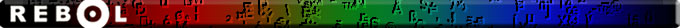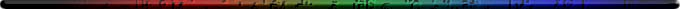Script Library: 1238 scripts

# Documentation for: parse-expression.r# Mathematical Expression Dialect Parser

Author: Francois Vanzeveren
Email: [rebol] [@] [rebol.x10.mx]
Web site: http://rebol.x10.mx

## Contents:

1. Introduction
2. Features
3. Precedence
4. Associativity (left and right)
5. Parentheses
6. Variables
7. Built-in functions
8. Signed numbers and signed expressions

## 1. Introduction

This is the documentation for parse-expression version 0.9.5 available on rebol.org.

This script is made of a single parse-expression function that takes a mathematical expression as a string! and translates it into a block of rebol code that can be evaluated with the do function.

```>> do %parse-expression.r
Script: "Mathematical Expression Dialect Parser" (15-Mar-2011)
>> expr: parse-expression "(1-x)**2/(1+x)"
== [divide power subtract 1.0 x 2.0 add 1.0 x]
>> x: 3
== 3
>> do expr
== 1.0
```

Could it be any easier?

Parse Equation or Parse Expression ?

Prior to version 0.9.4, parse-expression was named parse-equation. But Ladislav Mecir pointed out on altme this was a misuse of language. As a reminder:

 Equation Expression An equation is a SENTENCE. One solves an equation. An equation HAS a relation symbol. An expression is a PHRASE, a sentence fragment. One simplifies an expression. An expression HAS NO relation symbol.

As this parser handles expressions and not equations, starting with version 0.9.4, it has been renamed into parse-expression.

## 2. Features

• Supports the standard order of operations, or precedence:
1. terms inside brackets
2. exponents and roots
3. multiplication and division
• Handles left-associative ( + - * / ) and right-associative operators ( e.g. exponent)
• Supports unlimited level of nested parentheses
• Supports variables
• Supports trigonometric functions
• No syntax constraint regarding spaces between operands, operators, functions and variables
• Produces clean and straightforward rebol code

## 3. Precedence

There are five arithmetic operators: ** (exponentiation), * (multiplication), / (division), + (addition), and - (subtraction).

Exponentiation (**) is at the highest level of precedence, multiplication (*) and division (/) are at a lower level of precedence, and addition (+) and subtraction (-) are at an even lower level of precedence.

parse-expression conforms to the above standard precedence rules.

Let's start with a very simple example illustrating how precedence is (properly) managed:

```>> parse-expression "1+2*3"
== [add 1.0 multiply 2.0 3.0]
>> do parse-expression "1+2*3"
== 7.0
```

As you can see, the rebol code is build so as to first multiply 2 and 3 and then add the 1 to the result of the multiplication.

The two following examples mix the three levels of precedence and illustrate how the code is generated to first process exponentiation, then multiplication (division) and finally addition (subtraction).

```>> parse-expression "2-3**2/4"
== [subtract 2.0 divide power 3.0 2.0 4.0]
>> do parse-expression "2-3**2/4"
== -0.25

>> parse-expression "2/3**2-4"
== [subtract divide 2.0 power 3.0 2.0 4.0]
>> do parse-expression "2/3**2-4"
== -3.77777777777778
```

## 4. Associativity (left and right)

In mathematics the associativity specifies the order in which a sequence of operators of the same precedence are evaluated. Associativity can be left(-to-right) or right(-to-left).

• Addition, subtraction, mutiplication and division are left associative. In the absence of parentheses, addition (subtraction) and multiplication (division) is performed from left to right. Thus, the expression 1+3-4 is equivalent to (1+3)-4 and not 1+(3-4).
• The exponentiation operator is right-associative. In the absence of parentheses, exponentiation is performed from right to left. Thus, the expression 2**3**4 is equivalent to 2**(3**4) and not (2**3)**4

parse-expression conforms to the above standard associativity rules.

The first example illustrates the left-to-right associatity of the addition and subtraction:

```>> parse-expression "2-3+4-5+6"
>> do parse-expression "2-3+4-5+6"
== 4.0
>> parse-expression "(((2-3)+4)-5)+6"
>> do parse-expression "(((2-3)+4)-5)+6"
== 4.0
```

The second example illustrates the left-to-right associativity of the multiplication and division:

```>> parse-expression "2/3*4/5*6"
== [multiply divide multiply divide 2.0 3.0 4.0 5.0 6.0]
>> do parse-expression "2/3*4/5*6"
== 3.2
>> parse-expression "(((2/3)*4)/5)*6"
== [multiply divide multiply divide 2.0 3.0 4.0 5.0 6.0]
>> do parse-expression "(((2/3)*4)/5)*6"
== 3.2
```

The third example illustrates the right-to-left associativity of the exponentiation operator:

```>> parse-expression "2**3**4"
== [power 2.0 power 3.0 4.0]
>> do parse-expression "2**3**4"
== 2.41785163922926E+24
>> parse-expression "2**(3**4)"
== [power 2.0 power 3.0 4.0]
>> do parse-expression "2**(3**4)"
== 2.41785163922926E+24
```

## 5. Parentheses

You can override parse-expression's precedence rules by using parentheses to group expressions in the order that you wish parse-expression to evaluate them. Here are some examples where we use parentheses to force a different evaluation order than parse-expression would otherwise use.

2 * (3 + 4)

2^(3 * 4)

2/(3/3)

If you have any doubt, use parentheses to make sure parse-expression executes your expressions in the order in which you intend.

The first example illustrates how parentheses can be used to alter the standard precedence rules:

```>> parse-expression "2*3+4"
== [add multiply 2.0 3.0 4.0]
>> do parse-expression "2*3+4"
== 10.0
>> parse-expression "2*(3+4)"
== [multiply 2.0 add 3.0 4.0]
>> do parse-expression "2*(3+4)"
== 14.0
```

The second example illustrates how parentheses can be used to alter the standard associativity rules:

```>> parse-expression "2/3*4"
== [multiply divide 2.0 3.0 4.0]
>> do parse-expression "2/3*4"
== 2.66666666666667
>> parse-expression "2/(3*4)"
== [divide 2.0 multiply 3.0 4.0]
>> do parse-expression "2/(3*4)"
== 0.166666666666667
```

parse-expression supports unlimited level of nested parentheses.

## 6. Variables

parse-expression handles variables in mathematical expression as illustrated in the exemple below:

```>> expr: parse-expression "(1-x)**2/(1+x)"
== [divide power subtract 1.0 x 2.0 add 1.0 x]
```

The parsed expression can then be evaluated for any value of the variable(s):

```>> x: 3
== 3
>> do expr
== 1.0
```

Variable names

There is no restriction on variable names, but keep in mind that a variable will be a 'word in a block of rebol code. Therefore, if this 'word is a reserved word of the rebol language, this might cause conflicts and problems.

## 7. Built-in functions

parse-expression provides the following built-in functions:

• abs()
• arcos() or acos()
• arcsin() or asin()
• arctan() or atan()
• cos()
• exp()
• log() or log10()
• log2()
• ln()
• sin()
• sqrt()
• tan()

An insane example to illustrate built-in functions:

```>> parse-expression "3*log(0.25)**sqrt(3+cos(.25)/cos(.25))+3"
>> do parse-expression "3*log(0.25)**sqrt(3+cos(.25)/cos(.25))+3"
== 4.08742869947348
```

## 8. Signed numbers and signed expressions

parse-expression handles signed numbers and signed expression without any constraint.

```>> parse-expression "-(2*-5)/-sqrt(-8*2/(-2*--2))"
== [divide negate multiply 2.0 -5.0 negate square-root divide multiply -8.0 2.0 multiply -2.
0 negate -2.0]
>> do parse-expression "-(2*-5)/-sqrt(-8*2/(-2*--2))"
== -5.0
```MakeDoc2 by REBOL- 17-Mar-2011